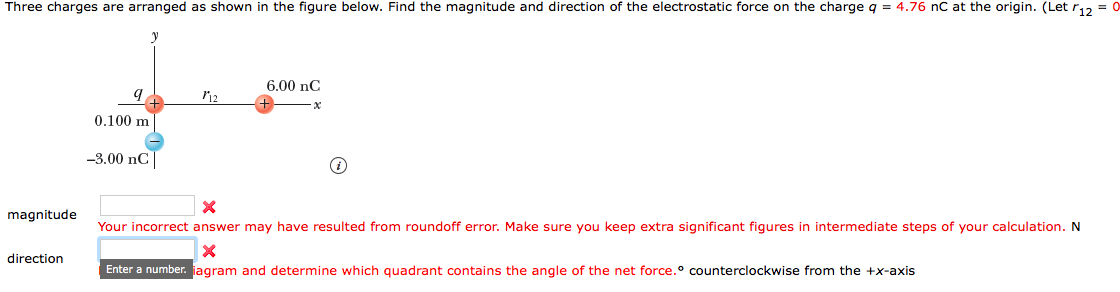# Three charges are arranged as shown in the figure below. Find the magnitude and direction of the electrostatic force on the charge q = 4.76 nC at the origin. (Let r12-0 6.00 nC 0.100 m -3.00 nC magnitude Your incorrect answer may have resulted from roundoff error. Make sure you keep extra significant figures in intermediate steps of your calculation. N direction Enter a number. jagram and determine which quadrant contains the angle of the net force.o counterclockwise from the +x-axis

Question

Three charges are arranged as shown in the figure below. Find the magnitude and direction of the electrostatic force on the charge q = 4.76 nC at the origin. (Let r12 = 0.240 m.)

Three point charges lie along the axes in the x y-coordinate plane.
Positive charge q is at the origin.
A charge of 6.00 nC is at (r1 2, 0), where r1 2 > 0.
A charge of −3.00 nC is at (0, −0.100 m).help_outlineImage TranscriptioncloseThree charges are arranged as shown in the figure below. Find the magnitude and direction of the electrostatic force on the charge q = 4.76 nC at the origin. (Let r12-0 6.00 nC 0.100 m -3.00 nC magnitude Your incorrect answer may have resulted from roundoff error. Make sure you keep extra significant figures in intermediate steps of your calculation. N direction Enter a number. jagram and determine which quadrant contains the angle of the net force.o counterclockwise from the +x-axis fullscreen

### Want to see the step-by-step answer?